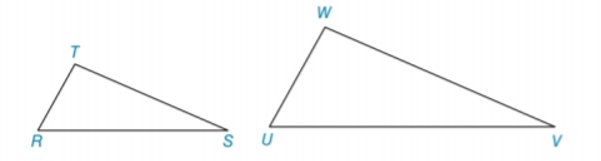Chapter 5.3, Problem 5EElementary Geometry For College St...

7th Edition
Alexander + 2 others
ISBN: 9781337614085

Solutions

Chapter
SectionElementary Geometry For College St...

7th Edition
Alexander + 2 others
ISBN: 9781337614085
Textbook Problem

In Exercises 5 to 8, name the method ( A A , S S S ~ ,   o r   S A S ~ ) that is used to show that the triangles are similar. W U = 3 2 · T R , W V = 3 2 · T S ,   a n d   U V = 3 2 · R STo determine

To name:

The method that is used to show that the triangles are similar.

Explanation

Given:

The triangles,

WU=32·TR,WV=32·TS, and UV=32·RS.

Approach:

Two polygons are similar if and only if two conditions are satisfied.

1. All pairs of corresponding angles are congruent.

2. All pairs of corresponding sides are proportional.

Definitions:

AA:

If the two angles of one triangle are congruent to the two angles of another triangle, then the triangles are similar.

SAS:

If an angle of one triangle is congruent to an angle of a second triangle and the pairs of sides including the angles are proportional (in length), then the triangles are similar.

SSS:

If the three sides of one triangle are proportional (in length) to the three corresponding sides of a second triangle, then the triangles are similar.

Description:

For the given triangle,

Still sussing out bartleby?

Check out a sample textbook solution.

See a sample solution

The Solution to Your Study Problems

Bartleby provides explanations to thousands of textbook problems written by our experts, many with advanced degrees!

Get Started

Find the slope of the line through P and Q. 8. P(1, 6), Q(4, 3)

Single Variable Calculus: Early Transcendentals, Volume I

What is the defining characteristic of a repeated-measures or within-subjects research design?

Essentials of Statistics for The Behavioral Sciences (MindTap Course List)

Sketch the graphs of the equations in Exercises 512. xy=2x3+1

Finite Mathematics and Applied Calculus (MindTap Course List)

Study Guide for Stewart's Multivariable Calculus, 8th

Evaluate 11(x2x3)dx a) 32 b) 56 c) 12 d) 23

Study Guide for Stewart's Single Variable Calculus: Early Transcendentals, 8th